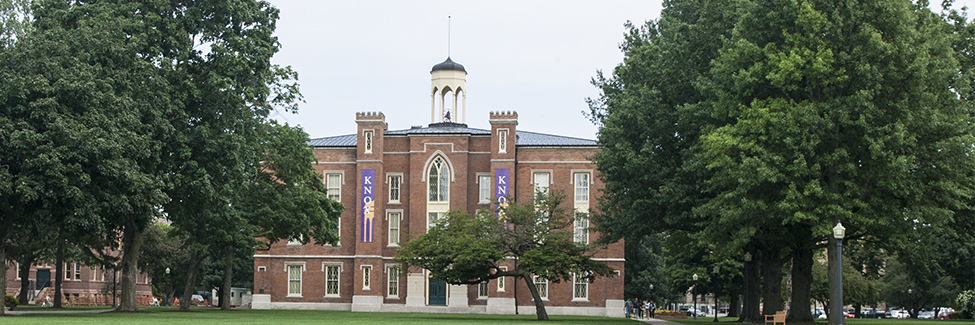Search# Requirements

Stuart Allison

Chair of Mathematics & Watson Bartlett Professor of Biology

Galesburg, IL 61401

309-341-7185

sallison@​knox.eduRequirements for the majors

Mathematics – Bachelor of Arts
11 credits as follows:

• Six core courses: MATH 152, MATH 185, MATH 205, MATH 231, MATH 235, MATH 241
• Electives: Four additional courses numbered 175 or higher, with at least one numbered above 300
• Research Experience: Each student must complete a research project leading to a written and oral presentation. This requirement may be fulfilled through MATH 361, MATH 399, or an honors project, and must be certified by the department chair.

Mathematics – Bachelor of Science
15 credits as follows:

• Eight core courses: MATH 152, MATH 185, MATH 205, MATH 230, MATH 231, MATH 235, MATH 241, MATH 321
• Electives. Four additional courses, with at least one numbered above 300, selected from the following list: MATH 215, MATH 217, MATH 225, MATH 313, MATH 322, MATH 325, MATH 331, MATH 332, MATH 333, MATH 341, MATH 342
• Research Experience. Each student must complete a research project leading to a written and oral presentation. This requirement may be fulfilled through MATH 361, MATH 399, or an honors project, and must be certified by the department chair.
• CS 141 or another programming course approved by the department chair.
• One additional course selected from the following list: BIOL 331, CHEM 321, CS 142, PHYS 130, or any full-credit Physics course numbered above 200

Requirements for the minor in Mathematics
5 credits as follows:

• MATH 152, MATH 185, MATH 205
• Two additional mathematics courses numbered 175 or higher, with at least one chosen from: MATH 216, MATH 217, MATH 218, MATH 231, MATH 235, MATH 241

Suggested coursework for various career paths

Mathematics majors intending to enter graduate school in Mathematics should complete all of MATH 215, MATH 230, MATH 313, MATH 331 and 333, and MATH 341 and 342.

Mathematics majors intending to pursue graduate study in Applied Mathematics should take all of MATH 215, MATH 230, MATH 321 and 322, MATH 331 and 333.

Students who are considering graduate study in Statistics should take a strong program in mathematics that includes MATH 151 and 152, MATH 185, MATH 205, MATH 235, MATH 321 and 322, and MATH 231 and 331.

Students interested in the actuarial profession should take introductory micro- and macroeconomics, MATH 151 and 152, MATH 185, MATH 205, MATH 227, MATH 235, and MATH 321 and 322.

Students who wish to teach at the secondary level should complete MATH 175, MATH 216, MATH 217, MATH 218, and MATH 321 and 322.